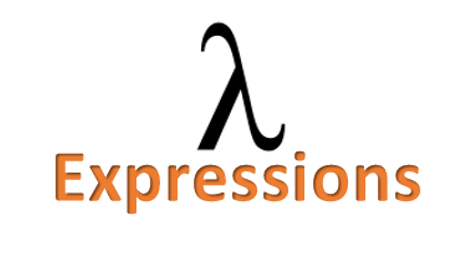# Java 8 Lambda Expressions

This article provides an introduction to lambda expressions introduced in JDK 8 and explains how to run them using functional interfaces.### What is a Lambda expression in Java ?

A Lambda expression represents an Anonymous method.

Anonymous method concept is similar to that of an Anonymous class… the difference being it implements a functional interface.

Functional interface is a new interface concept in Java 8. A functional interface can only declare one abstract method.

Lambda expressions allow the programmer to pass code in a concise manner making the code more clearer and flexible.

### Lambda syntax

A lambda expression contains:

• A parameter list
• An arrow symbol(->)
• Body of the lambda containing statements

The syntax for Lambda expressions is:

(parameters) -> {statements;}

### How to write simple methods as lambda expression

Here is a simple method that displays a message “Hello World”.

```public void hello(){
System.out.println("Hello World");
}
```

To convert this method to a lambda expression, remove the access specifier “public”, return type “void” and method name “hello” and write it as :
```() -> {System.out.println("Hello World");}
```

Here is another method example that adds two numbers and returns their sum :

```int add(int x, int y){
return x+y;
}
```

This method can be converted to lambda expression as :

```(int x, int y) -> {return x+y;}
```

### Important points about lambda expressions

Here are some notable points regarding lambda expressions :

#### 1 ) A lambda expression can have zero or more parameters.

Here is an example of lambda expression with zero parameters:

```() -> {System.out.println("Hello World");}
```

Here is an example of lambda expression with two parameters:

```(int x, int y) -> {return x+y;}
```

This lambda expression accepts two integer parameters and returns their sum.

#### 2) If the type of the parameters can be decided by the compiler, then we can ignore adding them in the lambda expression.

```(int x, int y) -> {return x+y;} // type mentioned

(x,y) -> {return x+y;}; // type ingored
```

#### 3) If there is only one parameter, the parenthesis of parameter can be omitted.

Here are some examples :

```x -> {return x+10;}

String s -> s.toUpperCase()

String s -> System.out.println(s)
```

#### 4) If the body has just one expression, the return keyword and curly braces can be omitted as show below:

```(int x, int y) -> x + y
```

If the return type of parameters is omitted, the compiler determines default parameter types.

```(x,y) -> x+y

(str, i) -> str.substring(i)
```

#### 5) A lambda can also have empty parameters

```() -> {return “Hello”;}

() -> System.out.println(“Hello”)
```

#### 6) A lambda can also have empty parameter as well as empty body

```() -> {}
```

This represents a valid lambda expression that takes no parameters and returns void.

### How to call a lambda expression ?

Once a lambda expression is written, it can be called and executed like a method.

For calling a lambda expression, we should create a functional interface.

Here is an example that uses functional interface to execute lambda expression :

```public class FuntionalInterfaceDemo {

interface MyInter{
void hello();
}
public static void main(String[] args) {
// TODO Auto-generated method stub
MyInter infVar = () -> {System.out.println("Hello World");};

infVar.hello();
}

}
```

Refer more details on functional interface and executing lambda expressions in the following article:

Running lambda expression with Functional Interface